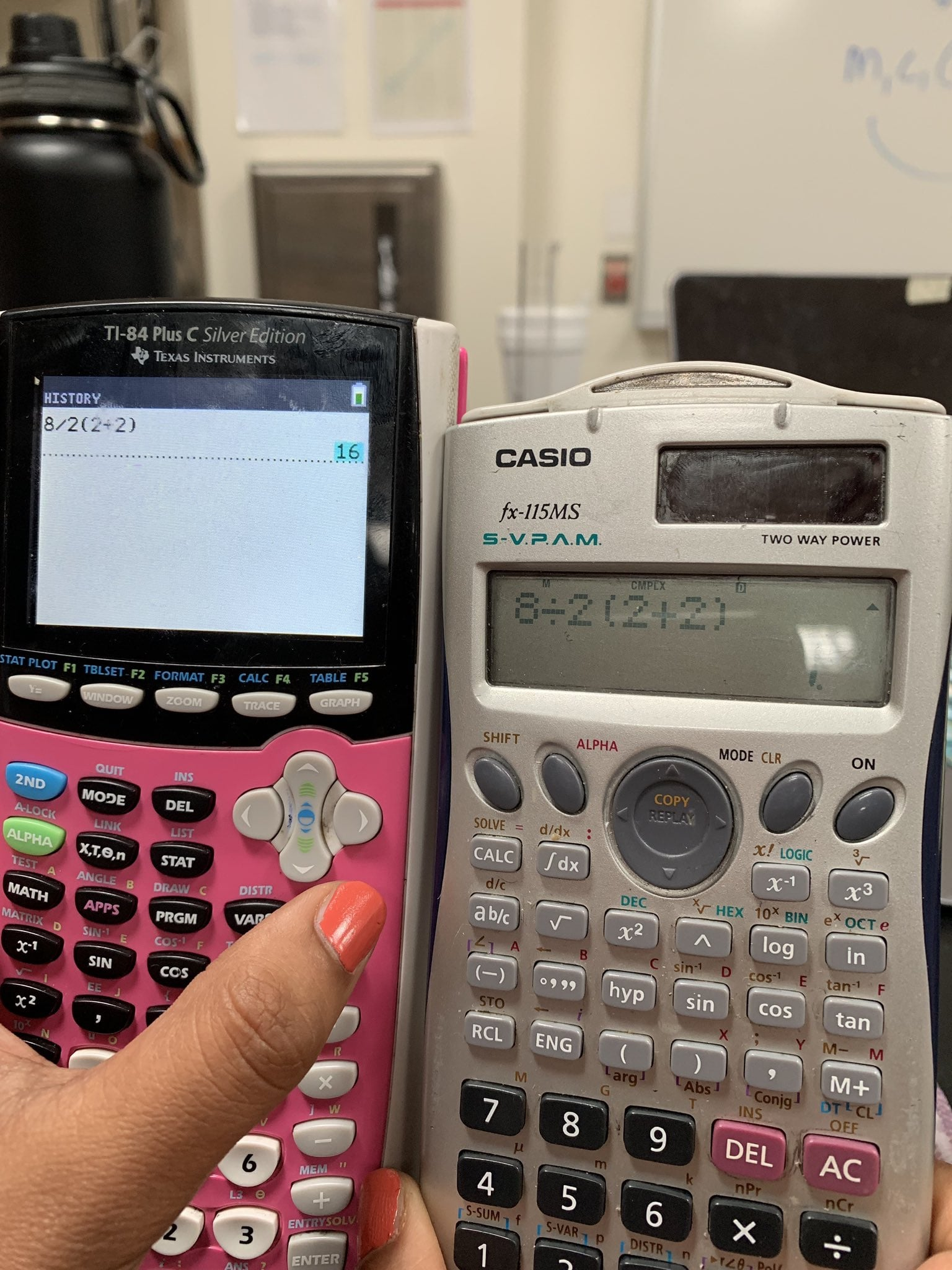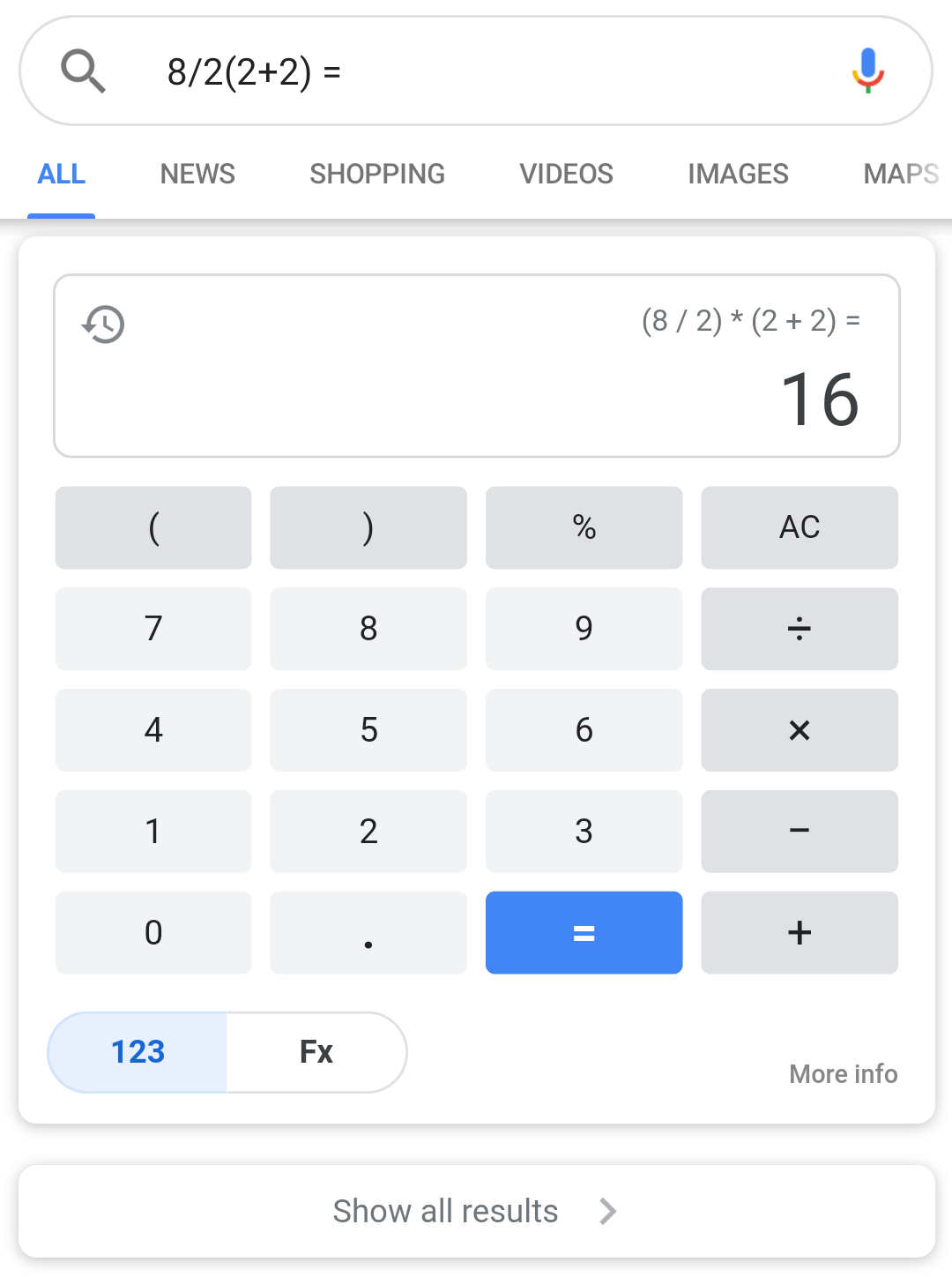#### Howdy, Stranger!

It looks like you're new here. If you want to get involved, click one of these buttons!Attention: Please take a moment to consider our terms and conditions before posting.

# 8/2(2+2) = ?

What we all need is another head scratcher over BODMAS

so here’s the latest offering sending the internet into arguments with strangers

8/2(2+2) = 1
or
8/2(2+2) = 16

even the calculators don’t agree...(pic from twitter, those nails are not my colour!!!)

.• Just put in brackets where it’s ambiguous and it’s easy.
• I'm saying 16. GCSE grade C.
• Texas Instruments (left) consider a fraction to be a separate function, so they multiply the [8/2] by [2+2], giving 16.
Casio (right) assume everything is part of the denominator unless otherwise stated, so it divides  by [2(2+2)], giving 1.

IMO, Casio is correct.
For Texas to be right, it should be written (8/2)(2+2). They’ve assumed an extra pair of brackets. But it’s open to interpretation which is why it’s not clear cut.

Bodmas says to do division and multiplication from left to right, but this expression isn’t clear enough about whether the [(2+2)] is on the top or the bottom of the fraction.

The whole problem occurs when both division and multiplication are involved and stems from the idea that in a fraction, the line separating the numerator from the denominator implies a pair of brackets around both the numerator and denominator. Which is why this probably should be 1, but you can see why some would say it’s 16.

Any mathematician worth his or her salt would write the expression as either
8/(2(2+2)) = 1
or
(8/2)(2+2) = 16
to avoid confusion.
• They’re both right. Pic on the left is multiplying a fraction, the pic on the right you have to sort the 2s first.
• It’s a poorly written equation that will always give two answers no matter which numbers you plug into it.
• Alternatively, the answer is 5! Right, @Chizz ?• For me it has to be 16.

If you look at the 8/2 as division it’s 4, just as it would be if you were looking at it as a fraction. The other way is if you rearrange an equation, the answer should still be the same. So if you have (2+2)8/2 it would be 16. You could also do (2+2) divide by 2 and multiply by the 8, it still comes out as 16.

That’s all I got.

•  Pringle said:
I'm saying 16. GCSE grade C.
It's 2+2 first which gives us 4 (as the brackets have been provided, you start with them), then 8/2 is 8 divided by 2 giving 4 and multiply them together
last. Which = 16

Also GCSE C so could well be wrong.

Just worked out that you could do

2+2

8/2 x 4

If the rule of always multiplying first is true it could be argued that you'd do 2x4 here so

8/8 = 1

• Via the PEDMAS rule it is 16 too.

D and M, and A and S is whichever is first. So once you have got the brackets out the way of (2+2) being 4. The next part of the equation is a division, which is 8/2, again being 4. The next part is the multiplication because of the open bracket, so 4x4=16.
• Sage gets 5! points

As soon as I saw pedmas it came rolling back!
• Chizz said:Do you get all the shit you post on here from Google Assistant?
• Divide and multiply do not take precedence over each other in any situation. Same with addition and subtraction.

PEDMAS/BODMAS should really be stylised as:

PEDA ... BODA
.....MS ........MS

to illustrate the above point better.

In that sense, you SHOULD add the two 2s together, then take care of the division and multiplication from left to right.

If you take the equation to mean 8 over 2(2+2) like a singular fraction then you’ll get 1.

In truth, if you were writing as a proper mathematician you’d know that there is an extra set of brackets missing and the equation should be written as either

a. (8/2)(2+2)=16
or
b. 8/(2(2+2))=1
•